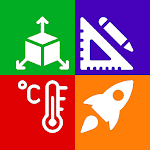ID: com.techsial.android.unitconverter

## The description of All in One Unit Converter Tool

Most of the times we need to convert different units and perform some scientific calculations such as to convert Length units we may need to convert inches to mm , convert inches to cm , convert inches to feet , convert cm to inches and to convert Weight units such as convert kg to lbs , convert lbs to kg or to convert Farenheit to Celcius etc

So, for Scientific calculations and to help you easily convert units, All in One Universal Unit Converter - Calculator is Engineering Unit conversion calculator which supports both Metric and Imperial Units and consists smart tools which are also very useful engineering tools. Following are units conversion categories under Conversions tab:

- Length / Distance Unit Converter (Kilometer to Mile or Foot to Inches etc)
- Area Units Converter (Hectare to Acre or Mile to Yard etc)
- Volume Unit Converter (Cubic Meter to Cubic centi meter etc)
- Mass / Weight Unit Converter (Kilogram to Pounds or Grams to Ounces etc)
- Cooking Unit Converter (Tea spoon to Table spoon or Cup to Tea spoon etc)
- Computer Storage Units Converter (Bit to Byte or Megabyte to Kilobyte etc )
- Temperature Units Converter (Fahrenheit to Celsius or Kelvin to Celsius etc)
- Time Unit Converter helps (Year to Month or Seconds to Nanoseconds etc)
- Fuel Units Converter (Kilometres per Litre to Miles per Litre etc)
- Speed Units Converter (Mile per hour to Kilometre per hour etc)
- Pressure Unit Converter (Kilopascal to Pascal or Torr to Pascal etc)
- Force Calculator using force formula ( Newton to Dyne etc)
- Torque Units Converter is to convert units used to measure torque
- Density Calculator using density formula
- Viscosity unit conversion
- Flow
- Surface Tension
- Energy Unit Converter (Joule to Calorie or Kilojoule to Joule etc)
- Power Units Converter (Megawatt to Kilowatt etc)
- Electric Current
- Electric Resistance of Resistor
- Electric Conductance
- Electrical Resistivity
- Electrical Conductivity
- Capacitance of Capacitor
- Inductance of Inductor
- Electric Charge Converter
- Electric Potential
- Sound Unit Converter
- Frequency conversion
- Screen Resolution

Unit Converter App also have very useful engineering tools which are implemented in Unit Converter - All in One Unit Conversion Tools to support and help in Engineering Unit Conversion. Following are these Smart Tools
under Tools tab:

- Scientific Calculator to perform Scientific calculations
- Stop Watch
- Memory Usage
- Protractor
- Periodic Table
- Number Counter
- Compass Needle
- Speed Meter
- RGB to HEX converter and HEX to RGB converter to convert RGB color codes to HEX color and vice versa
- Qibla Direction Finder
- Calendar
- Timer

For Scientific calculations following are math calculators under Calculations tab:

- Area Calculator
- Surface Area Calculator
- Perimeter Calculator
- Volume Calculator using volume formula
- Permutation Calculator
- Decimal to Fraction Calculator
- Proportion Calculator
- Date Difference Calculator (Age Calculator)
- Mileage Calculator , Distance Calculator , Fuel Calculator

Techsial Android Extreme Tech Arena is also offering:

- Find Device Info IMEI number - IMEI check ( https://play.google.com/store/apps/details?id=com.ghuman.apps.batterynotifier )
- Time Zone Converter - World Time Zones Clock ( https://play.google.com/store/apps/details?id=com.techsial.apps.timezones )

Contact: [email protected]
Connect with Techsial Android Extreme Tech Arena with: